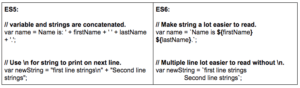Home » Features of ECMAScript 6 (ES6)

# Features of ECMAScript 6 (ES6)1. Variable:
• var (ES5): Variable scope is available within the function in which it is declared.
• let (ES6):
• let scope works only within the block in which it is declared like {}. be it function(){}, if(){} or just {}, etc.
• Does not allow redeclaring variable within the scope (Reduces unnecessary redeclaration of the same variable).
• const (ES6)
2. Parameters:
3. Template Literals:4. Arrow Functions:
5. Array:
6. String:
7. Maps:
8. Class Constructor and Methods:
9. Math:
• New methods have been added to the Math object.
• Math.sign: returns sign of a number as 1, -1 or 0.
Math.sign(5); // 1
Math.sign(-9); // -1
• Math.trunc: returns passed number without fractional digits.
Math.trunc(5.9); // 5
Math.trunc(5.123); // 5
• Math.cbrt: returns cube root of a number.
Math.cbrt(64); // 4
11. Destructuring:
12. Modules:
• It includes both a new syntax and a loader mechanism for modules.
// lib/math.js (create a math.js file in lib folder to export variable and methods.export function sum(x, y) {
return x + y;
}
export var pi = 3.141593;// app.js (create your app.js file to import methods/variable from module created above.
import { sum, pi } from “lib/math”;
console.log(‘2π = ‘ + sum(pi, pi));
13. Symbols:
• Symbols are a new primitive data type, like Number and String.
• It can be used to create unique identifiers for object properties or can create unique constants.
const MY_CONSTANT = Symbol();

I hope this post gives you some clear and simple idea of what is there in ES6. More can be read from below reference links:

References: